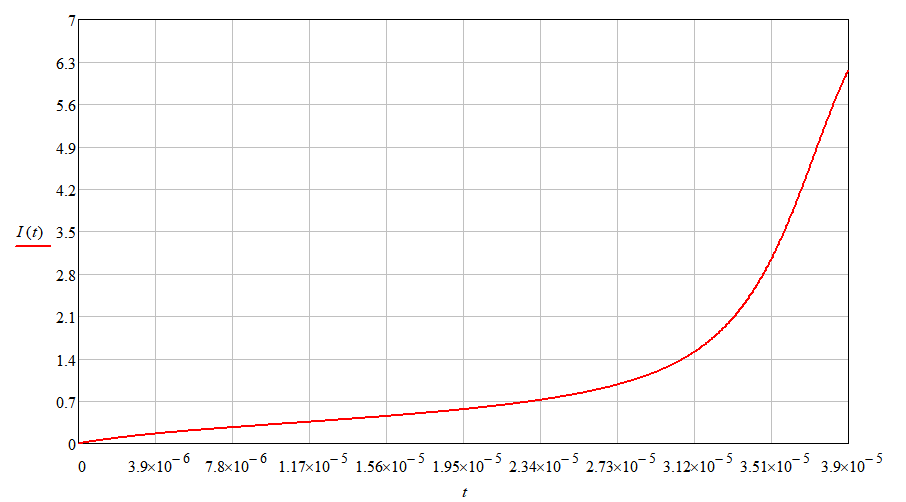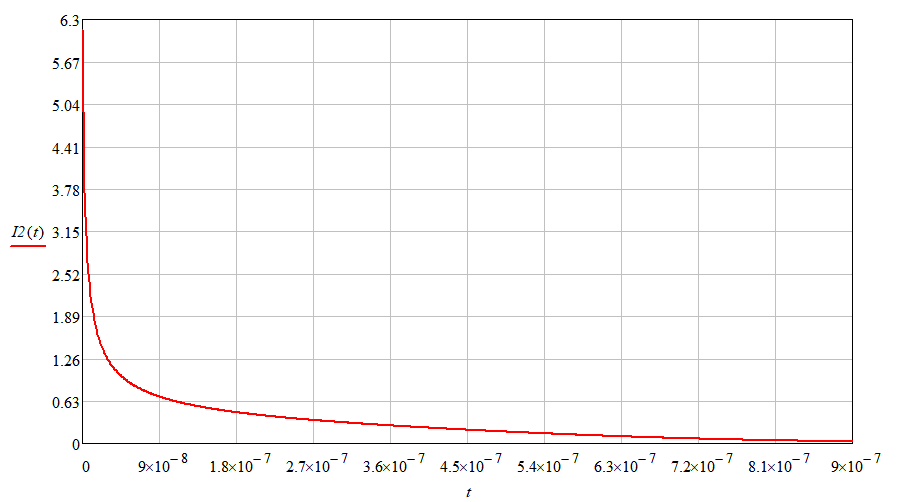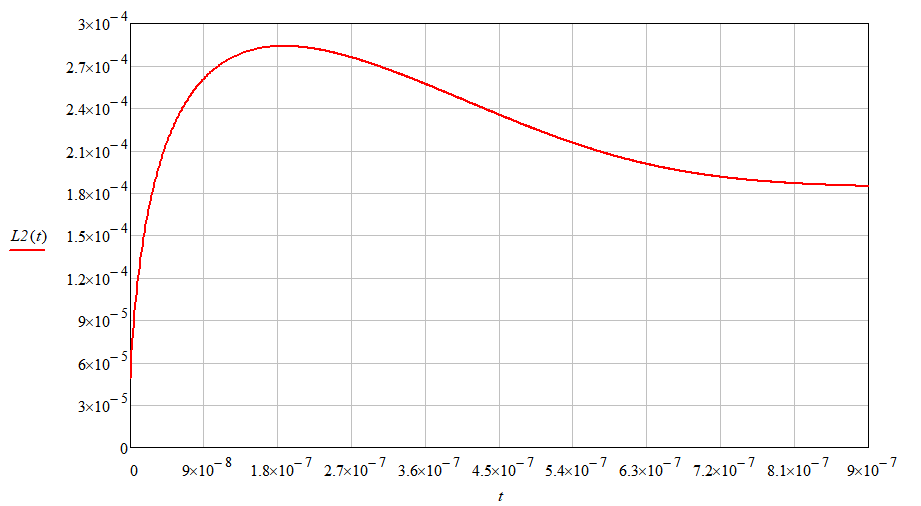Research website of Vyacheslav Gorchilin
2023-06-24
COP in an inductive parametric circuit of the second kind.
Parametric generators. In the part of this work, the author proposed a method for calculating the efficiency factor parametric chain of the second kind, based on the Stoletov curve. In the second part, we will gradually move from theory to practical developments, we will consider three methods for implementing this technology, and analyze the well-known free energy devices based on them. There will be fewer formulas and more descriptions of real processes. . Introduction to the Problem. From the analysis of parametric installations, starting from one of the first , we can conclude that that the cost of changing the initial inductance L0, usually fully corresponds to the possible energy increase. The mathematical logic here is as follows. At the first stage, we pump the inductor with current I1, spending energy on it: $W_1 = {L_0\, I_1^2 \over 2} \tag{2.1}$ Then we change the inductance to L1, and remove the following energy from this inductance: $W_2 = {L_1\, I_1^2 \over 2} \tag{2.2}$ Depending on whether the inductance has changed up or down, we get, in theory, a larger or smaller increase: $K_{\eta 2} = {W_2 \over W_1} = {L_1 \over L_0} \tag{2.3}$ Everything would be wonderful and we would extract energy simply from nothing, but nature is designed in such a way that so that such tricky methods of obtaining energy from it do not work, and the cost of changing the inductance would be equal to excess :). We have at our disposal only the curve of the change in inductance from the current in the coil. It's called the Stoletov curve, and can be represented as a simple : $L = L_0\, M\! \\M\! = {1 + k_{12} I^2 \over 0 + k_{22} I^2 + k_{23} I^3} + {1 \over \mu_i} \tag{2.4}$ where: μi is the initial relative magnetic permeability of the coil core, k12 k22 k23 - coefficients of the Stoletov curve, I is the current passing through the coil. At its core, this curve shows the hidden processes of reversal of magnetic domains in the ferromagnet of the core, depending on the current flowing in the coil. And if the dependence of the current on time is known, then the curve reveals the velocity and acceleration characteristics of the domain turn. We will use this property further. . But if we rely solely on nature, and we change the inductance of the coil only with the help of the current passing through it, then we get a parametric circuit of the first kind , and it, by its definition, cannot give an energy increase . To obtain excess energy, you will have to apply non-standard solutions, the general meaning of which is to change the characteristic M at different stages of the current passing through the coil. Then we can get a parametric chain , where such an increase becomes possible. . Devices with a similar principle of operation can be called parametric generators of the second kind . The method of changing the characteristic M in them is subdivided into electrical, semi-mechanical and mechanical. Depending on such a division, it is possible to determine the availability and complexity of preliminary calculations of generators. For example, the mechanical method requires measurements of M on a real working layout, which greatly complicates its calculation. For the electrical method, as a rule, it is sufficient to measure the change in this characteristic in a way represented by and . Let's start with him. . Electrical methods for changing M. The easiest way to get such a circuit is to divide the transient process into two stages: 0 - pumping the coil with current, 0 - picking up the energy pumped into the coil. Moreover, the movement of current at different stages should be divided into different circuits ; this is a necessary but not sufficient condition. At the first stage, the switch SW is closed, and the current, through the resistance R, flows into the coil L. After opening this switch, the current in the coil changes direction, which means that the polarity of the voltage on it becomes opposite and the current, at the second stage, flows through the D1 diode into the load resistance Rn. This is similar to flyback converter circuitry , but to obtain a parametric chain of the second kind from it, it is necessary to achieve different M, at different stages of the process. For the electric method of changing the inductance of the coil, an external influence is not required, which means that additional energy is not spent on it. . What does another characteristic M mean for a ferromagnet? After all, its magnetic domains are in their places, and their rotation around the axis is carried out in the same way. What then? The time of their rotation changes, which, obviously, depends on the rate of change of the magnetic field, which, in turn, depends on the voltage applied to the coil: the higher it is, the greater the growth rate of this field. There is a clear analogy with , the increase of which is directly proportional to the voltage. The growth of this parameter leads to an energy increase in the presented circuit, however, with the correct change in the M characteristics, at different stages of the transient process. .Let's analyze the consequence of changing the characteristic M for circuit 4a, in which the first stage of the transient process is carried out using a voltage source U=9 V through an active resistance R=1 Ohm. At this stage, the characteristic of the M1 coil is used, which has: k12=12.29, k22=2.16, k23=7.07. The current in the circuit I we get the same as in Figure 5. .The second stage passes through the diode D1 and the load resistance Rn=1000 Ohm, while using the characteristic M0 , where: k12=13.63, k22=3.55, k23=7.81, which is just slightly different from M1. The characteristics M were taken from a real coil with an initial inductance L0=0.18 mH, with a core with μ i=2000. The current in the second circuit I2 we get the same as in Figure 6. . We have already selected the optimal moment for switching the first stage of the transient process to the second, and it occurs at t=39 µs. Calculating the increase for this case using the formulas (), we get: Kη2=1.1. That is, Rn should release 10% more energy than consumed by the power source. At the same time, we should not forget that the efficiency of all elements of the device is not yet taken into account, which here will be determined mainly by losses in the SW key and D1 diode. . How to get different characteristics of M. This is the most important point of this technology. In contrast to the parametric change in the inductance, in which it must necessarily be less at the pumping stage and more at the removal stage, here this parameter can change in different directions, at different stages of the transient process. This is reflected in the following graphs, which are built on the basis of previous data . .